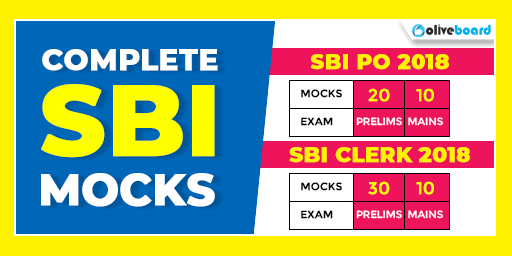# SBI PO 2018 Exam: Tips to Solve Number Series Questions in Less Time

SBI PO examinations are scheduled to be conducted in July and August. SBI PO 2018 Prelims would be conducted in July while SBI PO 2018 Mains would be conducted in August. Given that there is hardly 1 month left for the SBI PO Prelims and 2 for the SBI PO Mains, it is now the most important time of the year for your exam preparations. During the last few weeks before examinations, you must revise what you already know as well as brush up on what you find slightly tough. To help you prepare better, we have brought you an article on Number Series questions from the Quantitative Aptitude section.Number series questions are not particularly tough; however, they require lots of practice and can be quite time-consuming for the student to be successful in identifying the pattern in the question. However, given that they can be solved in just so many ways, this itself is a confusing aspect of number series questions, and in banking examinations, students need to be both fast and accurate. Therefore, to help you solve number series questions easily, we have come up with this blog article titled, ‘SBI PO 2018 Exam: Tips to Solve Number Series Questions in Less Time’. Use those tips to improve your accuracy at answering those questions.

• Number series questions require you to identify the wrong numbers in the series, for which you need to find out where the series’ pattern isn’t being followed and then single out those numbers.
• Try to memorize the squares and cubes of numbers from 1 – 20. This will help you identify patterns easily in case the number series includes the squares and/or cubes of those numbers (either separately or in combination with other fundamental patterns).
• There may be patterns based on the addition and subtraction of numbers, their multiplication and division, or their squares and/or cubes. Any other pattern would include a combination of two or more of those 3 fundamental patterns. You need to practice questions from each kind/category to be familiar with all of them.
• Pattern #1: Based on the addition and subtraction of numbers

Consider the number series: 3, 8, 13, 17, 23, 28, 33

If you are required to find the wrong number here which does not belong in the series, start by looking at the how the numbers add up or subtract from each other.

For example, 8 – 3 = 5, 13 – 8 = 5, 17 – 13 = 4, 23 – 17 = 6, 28 – 23 = 5, 33 – 28 = 5.  So, we get 5 when we subtract all numbers except for 17; when 17 is subtracted from 23, it gives a value of 6 and when 13 is subtracted from itself, the remainder is 4. Clearly, 17 violates the pattern. Hence, it’s the odd number in the series and the incorrect option.

• Pattern #2: Based on the multiplication and division of numbers

Consider the number series: 21, 63, 189, 567, 1800, 5400

One pattern that you may observe here is: 63 = 21+(21*2), 189 = 63+(63*2), 567 = 189+(189*2), but 1800 does not equal 567+(567*2) and that is the disruption of the order. Hence, the wrong number here is 1800 as it does not follow the pattern.

• Pattern #3: Based on the squares and cubes of numbers

Consider the number series: 27, 64, 216, 512, 1000, 1728, 2744

The pattern here is clear: they are all the cubes of numbers (which is evident from the fact that 64 is the cube of 4, 216 is the cube of 6, 512 is the cube of 8, etc.) However, they are all cubes, so what is the wrong number? Do note that all the numbers here are even except for 27 which is odd and that is because the cube root is 3, an odd number while all others are even numbers. Hence, 27 is the odd number here.

As you may have seen from the previous post, the main factor here is to ensure that the patterns are recognized correctly. However, given that there can be nearly infinite combinations of patterns, the only solution here is to practice more number of questions or rather, more kinds of questions from this topic.

So, keep working hard and do not give up. All the Best!